# Fraction calculator

The calculator performs basic and advanced operations with fractions, expressions with fractions combined with integers, decimals, and mixed numbers. It also shows detailed step-by-step information about the fraction calculation procedure. Solve problems with two, three, or more fractions and numbers in one expression.

## Result:

### 62/5 + 31/8 + 2/3 = 1223/120 = 10 23/120 ≅ 10.1916667

Spelled result in words is one thousand two hundred twenty-three one-hundred twentieths (or ten and twenty-three one-hundred twentieths).

### How do you solve fractions step by step?

1. Conversion a mixed number 6 2/5 to a improper fraction: 6 2/5 = 6 2/5 = 6 · 5 + 2/5 = 30 + 2/5 = 32/5

To find new numerator:
a) Multiply the whole number 6 by the denominator 5. Whole number 6 equally 6 * 5/5 = 30/5
b) Add the answer from previous step 30 to the numerator 2. New numerator is 30 + 2 = 32
c) Write a previous answer (new numerator 32) over the denominator 5.

Six and two fifths is thirty-two fifths
2. Conversion a mixed number 3 1/8 to a improper fraction: 3 1/8 = 3 1/8 = 3 · 8 + 1/8 = 24 + 1/8 = 25/8

To find new numerator:
a) Multiply the whole number 3 by the denominator 8. Whole number 3 equally 3 * 8/8 = 24/8
b) Add the answer from previous step 24 to the numerator 1. New numerator is 24 + 1 = 25
c) Write a previous answer (new numerator 25) over the denominator 8.

Three and one eighth is twenty-five eighths
3. Add: 32/5 + 25/8 = 32 · 8/5 · 8 + 25 · 5/8 · 5 = 256/40 + 125/40 = 256 + 125/40 = 381/40
For adding, subtracting, and comparing fractions, it is suitable to adjust both fractions to a common (equal, identical) denominator. The common denominator you can calculate as the least common multiple of both denominators - LCM(5, 8) = 40. In practice, it is enough to find the common denominator (not necessarily the lowest) by multiplying the denominators: 5 × 8 = 40. In the next intermediate step, the fraction result cannot be further simplified by canceling.
In words - thirty-two fifths plus twenty-five eighths = three hundred eighty-one fortieths.
4. Add: the result of step No. 3 + 2/3 = 381/40 + 2/3 = 381 · 3/40 · 3 + 2 · 40/3 · 40 = 1143/120 + 80/120 = 1143 + 80/120 = 1223/120
For adding, subtracting, and comparing fractions, it is suitable to adjust both fractions to a common (equal, identical) denominator. The common denominator you can calculate as the least common multiple of both denominators - LCM(40, 3) = 120. In practice, it is enough to find the common denominator (not necessarily the lowest) by multiplying the denominators: 40 × 3 = 120. In the next intermediate step, the fraction result cannot be further simplified by canceling.
In words - three hundred eighty-one fortieths plus two thirds = one thousand two hundred twenty-three one-hundred twentieths.

#### Rules for expressions with fractions:

Fractions - use the slash “/” between the numerator and denominator, i.e., for five-hundredths, enter 5/100. If you are using mixed numbers, be sure to leave a single space between the whole and fraction part.
The slash separates the numerator (number above a fraction line) and denominator (number below).

Mixed numerals (mixed fractions or mixed numbers) write as non-zero integer separated by one space and fraction i.e., 1 2/3 (having the same sign). An example of a negative mixed fraction: -5 1/2.
Because slash is both signs for fraction line and division, we recommended use colon (:) as the operator of division fractions i.e., 1/2 : 3.

Decimals (decimal numbers) enter with a decimal point . and they are automatically converted to fractions - i.e. 1.45.

The colon : and slash / is the symbol of division. Can be used to divide mixed numbers 1 2/3 : 4 3/8 or can be used for write complex fractions i.e. 1/2 : 1/3.
An asterisk * or × is the symbol for multiplication.
Plus + is addition, minus sign - is subtraction and ()[] is mathematical parentheses.
The exponentiation/power symbol is ^ - for example: (7/8-4/5)^2 = (7/8-4/5)2

#### Examples:

subtracting fractions: 2/3 - 1/2
multiplying fractions: 7/8 * 3/9
dividing Fractions: 1/2 : 3/4
exponentiation of fraction: 3/5^3
fractional exponents: 16 ^ 1/2
adding fractions and mixed numbers: 8/5 + 6 2/7
dividing integer and fraction: 5 ÷ 1/2
complex fractions: 5/8 : 2 2/3
decimal to fraction: 0.625
Fraction to Decimal: 1/4
Fraction to Percent: 1/8 %
comparing fractions: 1/4 2/3
multiplying a fraction by a whole number: 6 * 3/4
square root of a fraction: sqrt(1/16)
reducing or simplifying the fraction (simplification) - dividing the numerator and denominator of a fraction by the same non-zero number - equivalent fraction: 4/22
expression with brackets: 1/3 * (1/2 - 3 3/8)
compound fraction: 3/4 of 5/7
fractions multiple: 2/3 of 3/5
divide to find the quotient: 3/5 ÷ 2/3

The calculator follows well-known rules for order of operations. The most common mnemonics for remembering this order of operations are:
PEMDAS - Parentheses, Exponents, Multiplication, Division, Addition, Subtraction.
BEDMAS - Brackets, Exponents, Division, Multiplication, Addition, Subtraction
BODMAS - Brackets, Of or Order, Division, Multiplication, Addition, Subtraction.
GEMDAS - Grouping Symbols - brackets (){}, Exponents, Multiplication, Division, Addition, Subtraction.
Be careful, always do multiplication and division before addition and subtraction. Some operators (+ and -) and (* and /) has the same priority and then must evaluate from left to right.

## Fractions in word problems:

• How many 3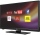How many hours the Andersons watched TV in all Wednesday 3/1 hr Thursdays 2/3 hr Friday 4/5 hr Saturday 3/4 hr
• Evaluate expressionEvaluate expression using BODMAS rule: 1 1/4+1 1/5÷3/5-5/8
• Complicated sum minus productWhat must be subtracted from the sum of 3/8 and 5/16 to get difference equal to the product of 5/8 and 3/16?
• A large 2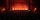A large popcorn bag holds four times as much as a small popcorn bag at the end of the party 3 1/3 small bags and 2 1/4 large bags left. How many small bags with the leftover popcorn fill?
• Evaluate 17Evaluate 2x+6y when x=- 4/5 and y=1/3. Write your answer as a fraction or mixed number in simplest form.
• Metal rodYou have a metal rod that’s 51/64 inches long. The rod needs to be trimmed. You cut 1/64 inches from one end and 1/32 inches from the other end. Next, you cut the rod into 6 equal pieces. What will be the final length of each piece?
• The cost 2The cost of 5 apples is \$3.45  and 5 oranges is \$1.23. If Rachel buys one apple and one orange, then how much must she pay?
• MarvinMarvin buys a hose that is 27 ¾ feet long. He already owns a hose at home that is ⅔ the length of the new hose. How many total yards of hose does Marvin have now?
• Baxter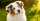Baxter ate 1/12 of a box of dog food. Now the box is 3/4 full. What fraction of the full of a full box was there before Baxter?
• Stock market 2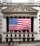For the week of July 22, the following day to day changes in the stock market was recorded a certain stock: -2 on Monday; +4 Tuesday; -8 Wednesday; + 2 1/2 Thursday; - 3 1/4 Friday. The stock began the week at 78 points. How many points did it finish with
• A 14.5-gallonA 14.5-gallon gasoline tank is 3/4 full. How many gallons will it take to fill the tank? Write your answer as a mixed number.
• Sayavong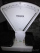Sayavong is making cookies for the class. He has a recipe that calls for 3 and 1/2 cups of flour. He has 7/8 of a cup of wheat flour, and 2 and 1/2 cups of white flour. Does Mr. Sayavong have enough flour to make the cookies?
• Food weightStacie is a resident at the medical facility where you work. You are asked to chart the amount of solid food that she consumes. For the noon meal, today she ate 1/2 of a 3-ounce serving of meatloaf, 3/4 of her 3-ounce serving of mashed potatoes, and 1/3 o